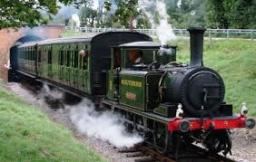# Passenger 2690

From city A to city B, the express train set off at an average speed of 100 km/h. At the same time, a passenger train departed from city B to city A. The trains met after 90 minutes. Calculate the average speed of a passenger train if the distance between the seats is 240 km.

v =  60 km/h

### Step-by-step explanation:Did you find an error or inaccuracy? Feel free to write us. Thank you!

Tips for related online calculators
Do you have a linear equation or system of equations and looking for its solution? Or do you have a quadratic equation?
Do you want to convert length units?
Do you want to convert velocity (speed) units?
Do you want to convert time units like minutes to seconds?